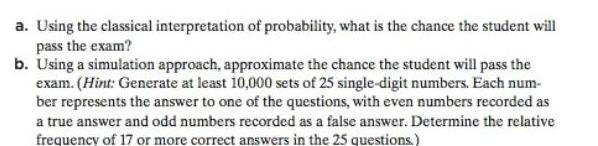Question:

# a Using the classical interpretation of probability what is

Last updated: 9/19/2023a Using the classical interpretation of probability what is the chance the student will pass the exam b Using a simulation approach approximate the chance the student will pass the exam Hint Generate at least 10 000 sets of 25 single digit numbers Each num ber represents the answer to one of the questions with even numbers recorded as a true answer and odd numbers recorded as a false answer Determine the relative frequency of 17 or more correct answers in the 25 questions